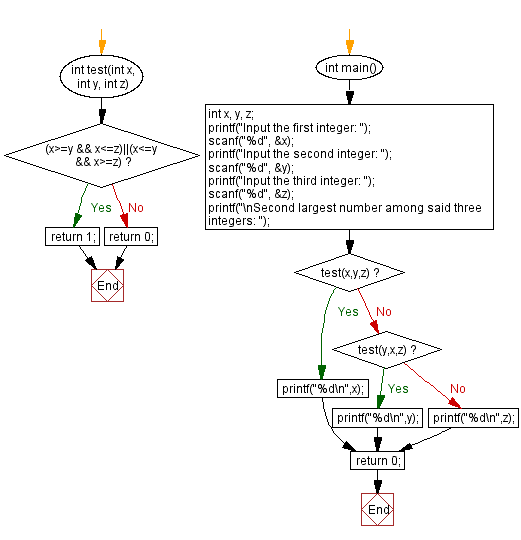﻿ C - Second largest among three integers

# C Exercises: Second largest among three integers

## C Basic-II: Exercise-3 with Solution

Write a C program that accepts three integers from the user and find second largest number among these.

Constraints:

• 1≤ x ≤100
• 1≤ y ≤100
• 1≤ z ≤100

Sample Date:

(1 , 2, 3) -> 2
(10, 12, 24) -> 12
(34, 21, 30) -> 30

C Code:

``````#include<stdio.h>
int test(int x, int y, int z)
{
if ((x>=y && x<=z)||(x<=y && x>=z))
return 1;
else
return 0;
}

int main()
{
int x, y, z;
printf("Input the first integer: ");
scanf("%d", &x);
printf("Input the second integer: ");
scanf("%d", &y);
printf("Input the third integer: ");
scanf("%d", &z);
printf("\nSecond largest number among said three integers: ");
if (test(x,y,z))
printf("%d\n",x);
else if (test(y,x,z))
printf("%d\n",y);
else printf("%d\n",z);
return 0;
}
``````

Sample Output:

```Input the first integer: Input the second integer: Input the third integer: 10, 12, 24
Second largest number among said three integers: 12
```

Flowchart:C Programming Code Editor:

Previous C Programming Exercise: Reverse a string partially.
Next C Programming Exercise: Common element(s) of two sequences.

What is the difficulty level of this exercise?

Test your Programming skills with w3resource's quiz.

﻿

## C Programming: Tips of the Day

C Programming - Execution time of C program:

CLOCKS_PER_SEC is a constant which is declared in . To get the CPU time used by a task within a C application, use:

```clock_t begin = clock();

/* here, do your time-consuming job */

clock_t end = clock();
double time_spent = (double)(end - begin) / CLOCKS_PER_SEC;
```

Note that this returns the time as a floating point type. This can be more precise than a second (e.g. you measure 4.52 seconds). Precision depends on the architecture; on modern systems you easily get 10ms or lower, but on older Windows machines (from the Win98 era) it was closer to 60ms.

clock() is standard C; it works "everywhere". There are system-specific functions, such as getrusage() on Unix-like systems.

Ref : https://bit.ly/2NFjFb7